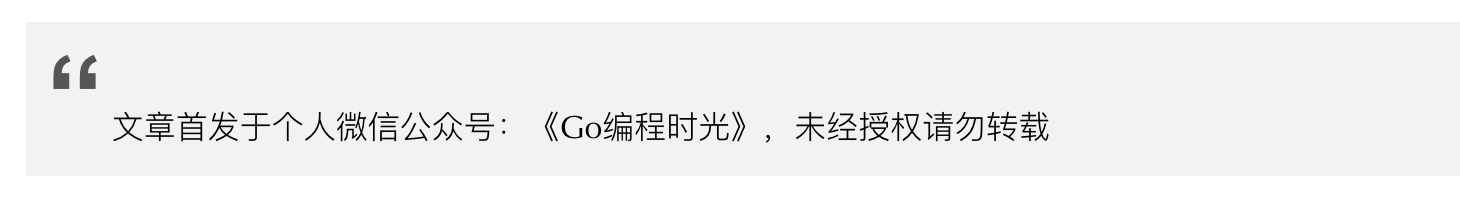# 7.2 Go 语言中边界检查¶## 1. 什么是边界检查？¶

```package main

func f(s []int) {
_ = s  // 检查第一次
_ = s  // 检查第二次
_ = s  // 检查第三次
}

func main() {}
```

```\$ go build -gcflags="-d=ssa/check_bce/debug=1" main.go
# command-line-arguments
./main.go:4:7: Found IsInBounds
./main.go:5:7: Found IsInBounds
./main.go:6:7: Found IsInBounds
```

## 2. 边界检查的条件？¶

```package main

func f() {
s := []int{1,2,3,4}
_ = s[:9]  // 不需要边界检查
}
func main()  {}
```

```package main

func f(s []int) {
_ = s[:9]  // 需要边界检查
}
func main()  {}
```

## 3. 边界检查的特殊案例¶

### 3.1 案例一¶

``` package main

func f(s []int) {
_ = s // 检查一次
_ = s  // 不会检查
_ = s  // 不会检查
}

func main() {}
```

### 3.2 案例二¶

```package main

func f(s []int) {
for index, _ := range s {
_ = s[index]
_ = s[:index+1]
_ = s[index:len(s)]
}
}

func main()  {}
```

### 3.3 案例三¶

```package main

import (
"math/rand"
)

func f()  {
s := make([]int, 3, 3)
index := rand.Intn(3)
_ = s[:index]  // 第一次检查
_ = s[index:]  // 不会检查
}

func main()  {}
```

```package main

import (
"math/rand"
)

func f()  {
s := make([]int, 3, 5)
index := rand.Intn(3)
_ = s[:index]  // 第一次检查
_ = s[index:]  // 第二次检查
}

func main()  {}
```

1. 当数组的长度和容量相等时，`s[:index]` 成立能够保证 `s[index:]` 也成立，因为只要检查一次即可

2. 当数组的长度和容量不等时，`s[:index]` 成立不能保证 `s[index:]` 也成立，因为要检查两次才可以

### 3.4 案例四¶

```package main

import (
"math/rand"
)

func f(s []int, index int) {
_ = s[:index] // 第一次检查
_ = s[index:] // 第二次检查
}

func main()  {}
```

```package main

import (
"math/rand"
)

func f(s []int, index int) {
_ = s[index:] // 第一次检查
_ = s[:index] // 不用检查
}

func main()  {}
```

## 5. 主动消除边界检查¶

```package main

func f(is []int, bs []byte) {
if len(is) >= 256 {
for _, n := range bs {
_ = is[n] // 每个循环都要边界检查
}
}
}
func main()  {}
```

```package main

func f(is []int, bs []byte) {
if len(is) >= 256 {
is = is[:256]
for _, n := range bs {
_ = is[n] // 不需要做边界检查
}
}
}
func main()  {}
```

## 参考文档¶